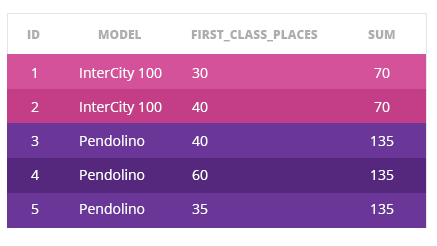Deals Of The Week - hours only!Up to 80% off on all courses and bundles.-Close
Introduction
PARTITION BY
5. PARTITION BY – Introduction
Summary

## Instruction

In this part, we'll learn one construction which can be put in OVER(), namely PARTITION BY. The basic syntax looks like this:

<window_function> OVER (PARTITION BY column1, column2 ... column_n)

PARTITION BY works in a similar way as GROUP BY: it partitions the rows into groups, based on the columns in PARTITION BY clause. Unlike GROUP BY, PARTITION BY does not collapse rows.

Let's see the example. For each train, the query returns its id, model, first_class_places and the sum of first class places from the same models of trains.With PARTITION BY, you can easily compute the statistics for the whole group but keep details about individual rows.

What functions can you use with PARTITION BY? You can use an aggregate function that you already know (COUNT(), SUM(), AVG(), etc.), or another function, such as a ranking or an analytical function that you'll get to know in this course. Within parentheses, in turn, we've now put PARTITION BY, followed by the columns by which we want to partition (group).

## Exercise

Run the template and see how it works.